[Next]: Stationary solutions of two-dimensional heterogeneous energy
 [Up]: Project descriptions
 [Previous]: Dissipative Schrödinger-Poisson systems
[Contents]   [Index]

## Physical modeling and numerical simulation of heat and carrier transport for high-power semiconductor devices semiconductor devices

Collaborator: H. Gajewski , H. Stephan

Cooperation with: G. Wachutka, W. Kaindl (Technische Universität München)

Supported by: DFG: Physikalische Modellierung und numerische Simulation von Strom- und Wärmetransport bei hoher Trägerinjektion und hohen Temperaturen'' (Physical modeling and numerical simulation of current and heat transport at high carrier injection and high temperatures) 

Description:

In 2002 our model , describing heat and carrier transport for semiconductor devices, has been advanced to silicon carbide (SiC). SiC is used in different crystal configurations (6H-SiC, 4H-SiC, 3C-SiC). Each of these materials possesses promising properties as basic materials for high-power, high-temperature and high-frequency electronics. The reason for this are special physical characteristics, which distinguish SiC from conventional semiconductor materials such as silicon. Those are first of all:

• Anisotropy: For each crystal configuration it is typical that physical quantities, such as carrier mobilities or heat conductivity, depend on the crystal orientation. It follows that appropriate physical quantities are no longer scalars but tensors. For example, the electron mobility in the hexagonal plain of 4H-SiC is five times larger than in the perpendicular direction.
• Wide band gap: The band gap in SiC is up to 3.2 eV (for comparison, the band gaps in Si and GaAS are 1.1 eV and 1.42 eV, respectively). This makes the material interesting for optoelectronics (blue LEDs). However, numerical problems can arise, since the gap enters exponentially into the equations of state. Additionally, the wide gap may lead to an only incomplete ionization of the dopants (in particular boron). This means, dopants have to be considered as traps.
• High temperature resistance: This--very welcome characteristic--requires the consideration of a large parameter area for temperature dependence of material data for the simulation.

Deriving the system of nonlinear partial differential equations for the heat and carrier transport in semiconductor devices, we abided by the following physical principles:

1.
postulation of the free energy density;
2.
calculation of the equilibrium according to the entropy maximum principle;
3.
definition of the thermodynamic potentials near the equilibrium state;
4.
postulation of the evolution equations;
5.
calculation of the currents and right-hand sides according to the second law of thermodynamics;
6.
derivation of the heat equation.

The postulated system of equations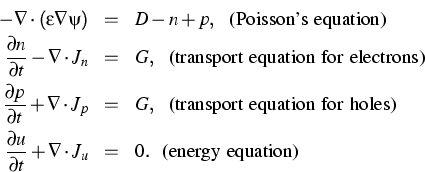describes electron, hole and energy transfer, which is nonlinearly coupled by the electrostatic potential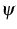via Poisson's equation. Here, n, p and u are electron, hole and power density, Jn, Jp and Ju the appropriate currents, D the dopants and G the generation-recombination rate. (The ODEs describing the dynamics of electron/hole traps were described in detail in the WIAS Annual Research Report 1998.)

The determination of the equilibrium as state of maximal entropy by Lagrange's method suggests the Lagrange multipliers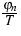,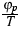and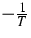to be thermodynamic potentials. Their gradients are the driving forces for the currents. That leads to the following current, under consideration of Onsager's principle: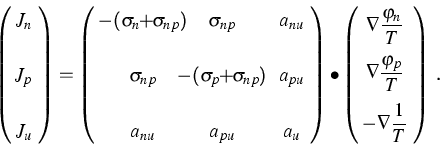In the case of the anisotropic SiC,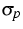,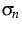and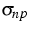, and anu, anp and au are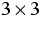matrices. From the second law of thermodynamics (entropy S increasing in time)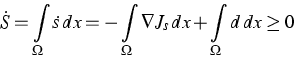(here d denotes the dissipation rate) it follows for the currents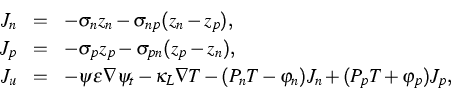with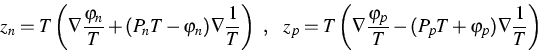and the energy carrier interaction terms (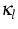is the heat conductivity)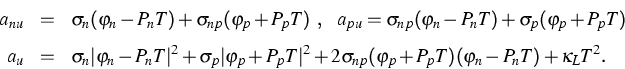For this model, thermodynamically consistent algorithms were developed and implemented into our program system WIAS-TeSCA .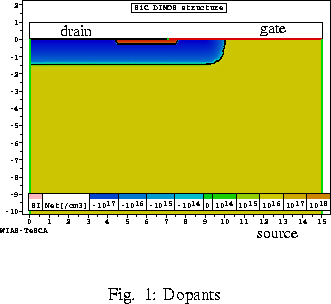As an example we show a 6H-SiC DIMOS transistor--a typical high-power device (). The crystal is oriented in such a way that the electron mobility in horizontal direction is five times higher than in the vertical direction. Figure 2 shows the electron flow for a gate voltage of 12 V and a drain voltage of 30 V. In comparison, Figure 3 shows the simulation result for isotropic mobility (e.g., in Si).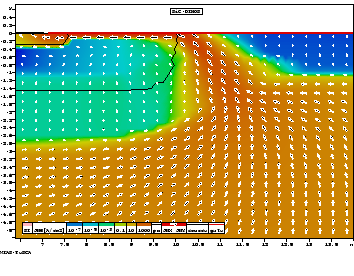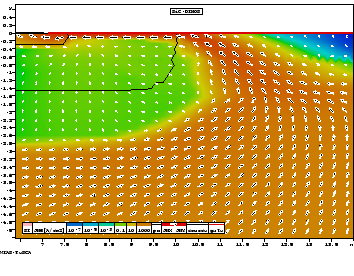References:

1. G. ALBINUS, H. GAJEWSKI, R. HÜNLICH, Thermodynamic design of energy models of semiconductor devices, Nonlinearity, 16 (2002), pp. 367-383.
2. H. GAJEWSKI, G. WACHUTKA, DFG-Antrag zum Projekt Physikalische Modellierung und numerische Simulation von Strom- und Wärmetransport bei hoher Trägerinjektion und hohen Temperaturen'', 1996, 1998, 2000 (applications for a DFG project).
3. H. GAJEWSKI, Analysis und Numerik des Ladungsträgertransports in Halbleitern, GAMM-Mitteilungen, 16 (1993), pp. 35-57.
4. M. LADES, Modelling and simulation of wide bandgap semiconductor devices: 4H/6H-SiC, Shaker Verlag, Aachen 2002.
5. G. WACHUTKA, Rigorous thermodynamic treatment of heat generation and conduction in semiconductor device modeling, IEEE Trans. on CAD, CAD-9 (1990), pp. 1141-1149.

 [Next]: Stationary solutions of two-dimensional heterogeneous energy
 [Up]: Project descriptions
 [Previous]: Dissipative Schrödinger-Poisson systems
[Contents]   [Index]

LaTeX typesetting by I. Bremer
5/16/2003